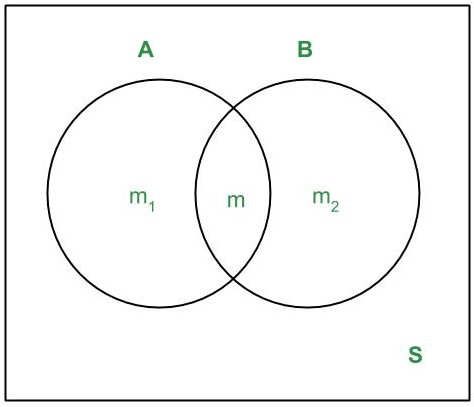GeeksforGeeks App
Open AppBrowser
Continue

# Multiplication Theorem

Probability refers to the extent of the occurrence of events. When an event occurs like throwing a ball, picking a card from the deck, etc ., then there must be some probability associated with that event. In terms of mathematics, probability refers to the ratio of wanted outcomes to the total number of possible outcomes. The probability of simultaneous occurrence of two events A and B is equal to the product of the probability of the other, given that the first one has occurred. This is called the Multiplication Theorem of probability.

## The Theorems

### or, P(A ∩ B) = P(B)P(A/B)    if P(B) ≠ 0

Proof:

Let S be the sample space associated with the given random experiment. Suppose S contains n elementary events. Let m1,m2 and m be the number of elementary events favorable to A,B and A ∩ B respectively, Then,

P(A) = m1/n, P(B) = m2/n and P(A∩B) = m/n

Since m1 elementary events are favorable to A out of which m are favorable to B. Therefore, P(B/A) = m/m1.                           Similarly, we have P(A/B) = m/m2.Now,    P(A ∩ B) = m/n

or,        P(A ∩ B) = m/m1 × m1/n = P(B/A) × P(A)    … (i)

Again,  P(A ∩ B) = m/n

or,        P(A ∩ B) = m/m2 × m2/n = P(A/B) × P(B)     …(ii)

Note 1:

From (i) and (ii) in the above theorem, we obtain that

P(B/A) = P(A ∩ B)/P(A) and P(A/B) = P(A ∩ B)/P(B)

Prove That: For P(B) > 0, P(A/B) ≤ P(A)

Proof:

n( A ∩ B) ≤ n(A) and n(B) ≤ n(S)

Dividing, we get n( A ∩ B) / n(B) ≤ n(A)/ n(S)

=> P(A/B) ≤ P(A)

Note 2:

(i) The conditional probability P(A/B) is not defined if P(B) = 0.

(ii) P(B / B) = 1

Remark: If A and B are independent events, then

P(A/B) = P(A) and P(B/A) = P(B)

∴  P(A ∩ B) = P(A) P(B)

### where P(Ai / A1 ∩ A2 ∩ … ∩ Ai-1) represents the conditional probability of the occurrence of event Ai, given that the events  A1, A2, …, Ai-1 have already occurred.

Particular Case: If A, B, C are three events associated with a random experiment, then

P(A ∩ B ∩ C) = P(A) P(B/A) P(C/A ∩ B)

### P(A ∩ B) = P(A) . P(B)

Proof:

We have: P(A ∩ B) = P(A) P(B/A) = P(B) P(A/B); P(A) ≠ 0  . . . (i)

If A and B are independent, i.e A is independent of B and B is independent of A, then, we have

P(A/B) = P(A) and P(B/A) = P(B) . . . (ii)

From (i) and (ii), we get: P(A ∩ B) = P(A) P(B), as required.

### Examples

Example 1: Find the probability of drawing a diamond card in each of the two consecutive draws from a well-shuffled pack of cards, if the card drawn is not replaced after the first draw?

Solution:

Let A be the event of drawing a diamond card in the first draw and B be the event of drawing a diamond card in the second draw. Then,

P(A) = 13C1 / 52 C1 = 13/52 = 1/4

After drawing a diamond card in the first draw 51 cards are left out of which 12 cards are diamond cards.

∴ P(B/A) = Probability of drawing a diamond card in the second draw when a diamond card has already been drawn in the first draw

P(B/A) = 12C1 / 51C1 = 12/51 = 4/17

Required probability = P(A ∩ B) = P(A) P(B/A) = 1/4 × 4/17 = 1/17

Example 2: A bag contains 19 tickets, numbered from 1 to 19. A ticket is drawn and then another ticket is drawn without replacement. Find the probability that both tickets will show even numbers.

Solution:

Let A be the event of drawing an even numbered ticket in the first draw and B be the event of drawing an even numbered ticket in the second draw. Then,

Required probability = P(A ∩ B) = P(A) P(B/A)   … (i)

Since there are 19 tickets, numbered 1 to 19, in the bag out of which 9 are even numbered viz. 2, 4, 6, 8, 10, 12, 14, 16, 18.

∴ P(A) = 9/19

Since the ticket drawn in the first draw is not replaced, therefore second ticket drawn is from the remaining 18 tickets, out of which 8 are even numbered.

∴ P(B/A) = 8/18 = 4/9

Substituting these values in (i), we get

Required probability = P(A ∩ B) = P(A) P(B/A) = 9/19 × 4/9 = 4/19

Example 3: It is 8:5 against the wife who is 40 years old living till she is 70 and 4:3 against her husband now 50 living till he is 80. Find the probability that

(i) Both will be alive,

(ii) None will be alive,

(iii) Only wife will be alive,

(iv) Only husband will be alive

Solution:

Let us define the events:

A: Wife will be alive,

B: Husband will be alive

Then, we are given:

P(A) = 5/(8 + 5) = 5/13 => P(A’) = 1 – P(A) = 8/13

P(B) = 3/(4 + 3) = 3/7  => P(B’) = 1 – P(B) = 4/7

If we assume that A and B are independent so that A and B’, A’ and B’ are also independent , then the required probabilities are given by:

(i) P(A ∩ B) = P(A)P(B) = (5/13) × (3/7) = 15/91

(ii) P(A’ ∩ B’) = P(A’)P(B’) = (8/13) × (4/7) = 32/91

(iii) P(A ∩ B’) = P(A) – P(A ∩ B) = (5/13) – (15/91) = 20/91

(iv) P(A’ ∩ B) = P(B) – P(A ∩ B) = (3/7) – (15/91) = 24/91

My Personal Notes arrow_drop_up
Related Tutorials